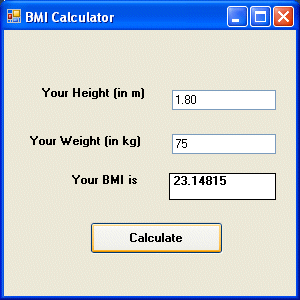# Visual Basic 2012 Lesson 7- Mathematical Operations

#### [Lesson 6] >> [CONTENTS] >> [Lesson 8]

In Visual Basic 2012, we can write code to instruct the computer to perform mathematical operations. To write code for mathematical operations, we need to use arithmetic operators. Visual Basic 2012 arithmetic operators are very similar to the normal arithmetic operators, only with little variations. The plus and minus operators are the same while the multiplication operator uses the * symbol and the division operator uses the / symbol. The list of Visual Basic 2012 arithmetic operators is shown in Table 7.1.

#### Table 7.1

Operator Mathematical Function Example
Subtraction  10-4=6
^  Exponential  3^2=9
*  Multiplication  5*6=30
/  Division  21/7=3
Mod  Modulus(returns the remainder of an integer division)  15 Mod 4=3
\  Integer Division(discards the decimal places)  19/4=4

#### Example 7.1

In this program, insert two Textboxes, four labels, and a button. Click the button and enter the code as shown below.  When you run the program, it will perform the four basic arithmetic operations and displays the results on the four labels.

```Private Sub Button1_Click(ByVal sender As System.Object, ByVal e As System.EventArgs) Handles Button1.Click
Dim num1, num2, difference, product, quotient As Single
num1 = TextBox1.Text
num2 = TextBox2.Text

sum=num1+num2
difference=num1-num2
product = num1 * num2
quotient=num1/num2
Label1.Text=sum
Label2.Text=difference
Label3.Text = product
Label4.Text = quotient

End Sub
```

#### Example 7.2

The program can use Pythagoras Theorem to calculate the length of hypotenuse c given the length of the adjacent side a and the opposite side b. In case you have forgotten the formula for the Pythagoras Theorem, it is written as
c^2=a^2+b^2

```Private Sub Button1_Click(ByVal sender As System.Object, ByVal e As System.EventArgs) Handles Button1.Click
Dim a, b, c As Single
a = TextBox1.Text
b = TextBox2.Text
c=(a^2+b^2)^(1/2)
Label3.Text=c
End Sub
```

#### Example 7.3: BMI Calculator

A lot of people are obese now and it could affect their health seriously. Obesity has proven by the medical experts to be one of the main factors that bring many adverse medical problems, including the cardiovascular disease. If your BMI is more than 30, you are considered obese. You can refer to the following range of BMI values for your weight status.

Underweight = <18.5
Normal weight = 18.5-24.9
Overweight = 25-29.9
Obesity = BMI of 30 or greater

To calculate your BMI, you can create a VB BMI calculator. The BMI calculator can calculate the body mass index or BMI of a person based on the body weight in kilogram and the body height in meter. BMI can be calculated using the formula weight/( height )^2, where weight is measured in kg and height in meter. If you only know your weight and height in lb and feet, then you need to convert them to the metric system (you could indeed write a VB program for the conversion).

```Private Sub Button1_Click(ByVal sender As System.Object, ByVal e As System.EventArsgs) Handles Button1.Click
Dim height, weight, bmi As Single
height = TextBox1.Text
weight = TextBox2.Text
bmi = (weight) / (height ^ 2)
Label4.Text = bmi
End Sub
```

The output is shown in the figure below. In this example, your height is 1.80m( about 5 foot 11), your weight is 75 kg( about 168Ib), and your BMI is about 23.14815. The reading suggests that you are healthy. (Note; 1 foot=0.3048, 1 lb=.45359237 kilogram)From the above examples, you can see that writing code that involve arithmetic operations is relatively easy. Here are more arithmetic projects you can try to program:

Area of a triangle
Area of a rectangle
Area of a circle
Volume of a cylinder
Volume of a cone
Volume of a sphere
Compound interest
Future value
Mean
Variance
Sum of angles in polygons
Conversion of lb to kg
Conversion of Fahrenheit to Celsius